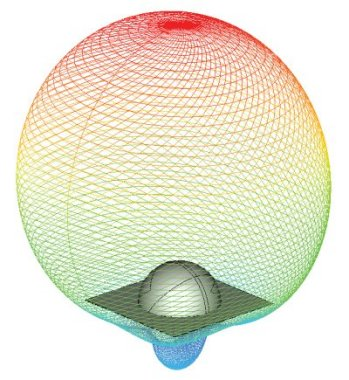APPLICATIONS

### Antenna Radiation Characteristics

CHRONOS™ and SINGULA™ successfully compute the radiation characteristics of antennas of different types: linear antennas, microstrip antennas, dielectric resonator antennas, horn antennas, reflector antennas and others.CHRONOS™ can calculate the radiation characteristics at many frequencies within the frequency band of the input transient pulse from a single run.

Our software can accurately calculate far zone radiation patterns of different components of electric and magnetic fields in the form of a 2D polar plot, a 2D rectangular plot or a full 3D plot. SINGULA™ calculates precisely the phase centre, input impedance and radiation efficiency of an antenna. The program also determines the power loss in a dielectric volume or the ohmic loss in a conducting volume.

CHRONOS™ and SINGULA™ analyze the effect of the presence of thin dielectric surfaces and/or thick dielectric volumes, arbitrarily shaped objects of finite and/or infinite conductivities on the radiation characteristics. Polarization characteristics such as axial ratio and tilt angle plots as a function of observation angle can be easily obtained.